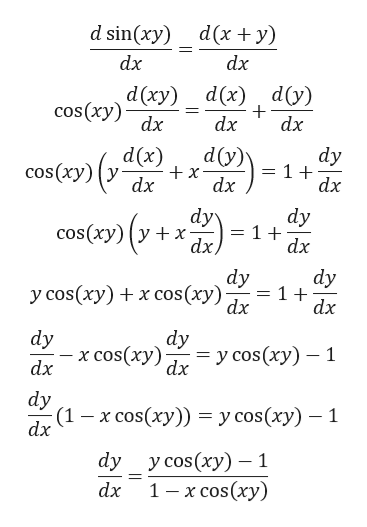# find the implicit formula of sinxy=x+y

Question

find the implicit formula of sinxy=x+y

check_circleExpert Solution
Step 1

Given,

Step 2

Differentiating on both side wi...help_outlineImage Transcriptionclosed sin(xy) d(x y) dx dx dy) d(x) d (ху) cos (xy) dx dx dx d(y) dy 1 + dx d(x) cos(xy) y dx x dx dy dy = 1 + dx olу cos(xy) y x- dx dy 1 + dx dy у cos (ху) + x соs (ху) dx dy dy х cos(ху) —у сos(ху) — 1 dx dy (1— х сos(ху)) %3 усos(ху) — 1 dx у cos (ху) — 1 1 — х cos(ху) dy dx fullscreen

### Want to see the full answer?

See Solution

#### Want to see this answer and more?

Solutions are written by subject experts who are available 24/7. Questions are typically answered within 1 hour*

See Solution
*Response times may vary by subject and question
Tagged in

### Derivative